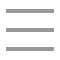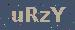个人随笔

2021-08-26 22:24:17

### 分析

#### 3、怎么找到状态转移方程？

1、最优子结构
2、重叠子问题

``dp(n)=dp(n-1)+dp(n-2):dp(1)=1;dp(2)=2``

### 解题

#### 1、粗暴递归

``public int op(int n) {``        if(n==1) {``            return 1;``        }``        if(n==2) {``            return 2;``        }``        //1、当前阶梯是上一阶梯爬一步上来的``        int one = op(n-1);``        //2、当前阶梯是上一阶梯爬两步上来的``        int two = op(n-2);``        return one+two;``    }``

#### 2、动态规划的方式解决

``public int climbStairs(int n) {``        //dp函数是一个以为数组，存放的是阶梯n对应的方法数目``        int[] dp = new int[n];``        if(n>=1) {``            dp=1;``        }``        if(n>=2) {``            dp=2;``        }``        for(int i=2;i<n;i++) {``            dp[i]=dp[i-1]+dp[i-2];``        }``        return dp[n-1];``    }``

``public int climbStairs2(int n) {``        //dp函数是一个以为数组，存放的是阶梯n对应的方法数目``        if(n==1) {``            return 1;``        }``        if(n==2) {``            return 2;``        }``        int one=1;``        int two=2;``        int now=0;``        for(int i=2;i<n;i++) {``            now=one+two;``            one=two;``            two=now;``        }``        return now;``    }``

### 总结

1、判断问题是否具有【最优子结构】，也就是子问题和子问题之间不会互相影响
2、判断问题是否具有【重叠子问题】，毕竟动态规划的本质就是穷举，只不过把子问题的解缓存了起来而已
3、确定【状态】，也就是原问题和子问题中会变化的变量，比如零钱找零问题的状态就是金额，爬楼梯问题的状态就是楼梯数目
4、确定【选择】，也就是导致状态变化的量，比如选择爬1还是爬2
5、确定【状态函数】，一般参数会包含状态，结果为目标值
6、确定【基准值】，也就是状态为0或者1或者2的时候，函数的值
7、列出【状态转移方程】
8、编码解决【缓存子问题结果

``# 初始化 base case``dp[...] = base``# 进行状态转移``for 状态1 in 状态1的所有取值：``    for 状态2 in 状态2的所有取值：``        for ...``            dp[状态1][状态2][...] = 求最值(选择1，选择2...)``
128Copyright : 个人随笔   备案号 : 粤ICP备18099399号